Homework Help Question & Answers

# Need some assistance of reorganizing this whole program. I have the right code for everything I...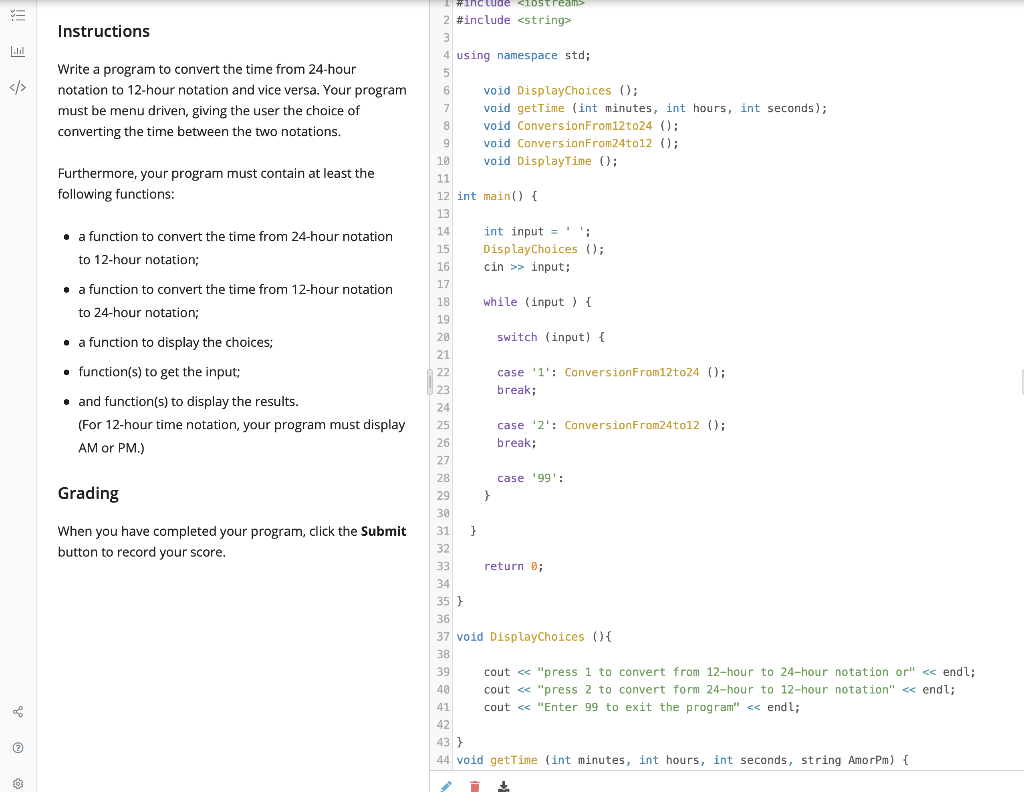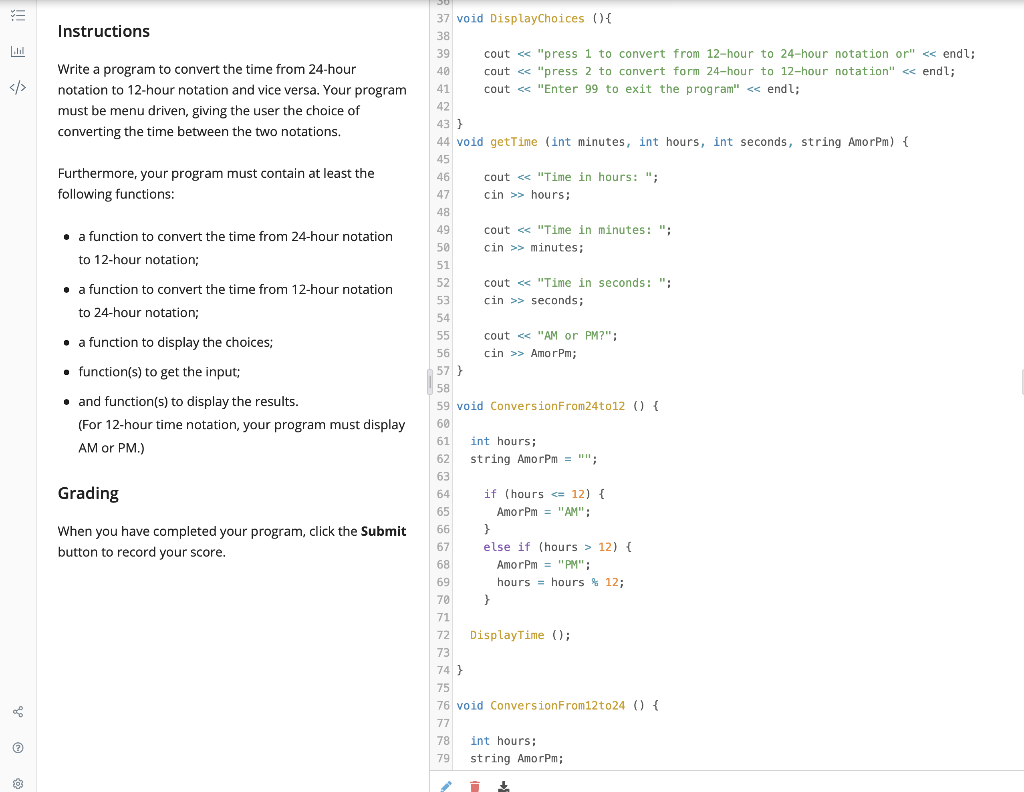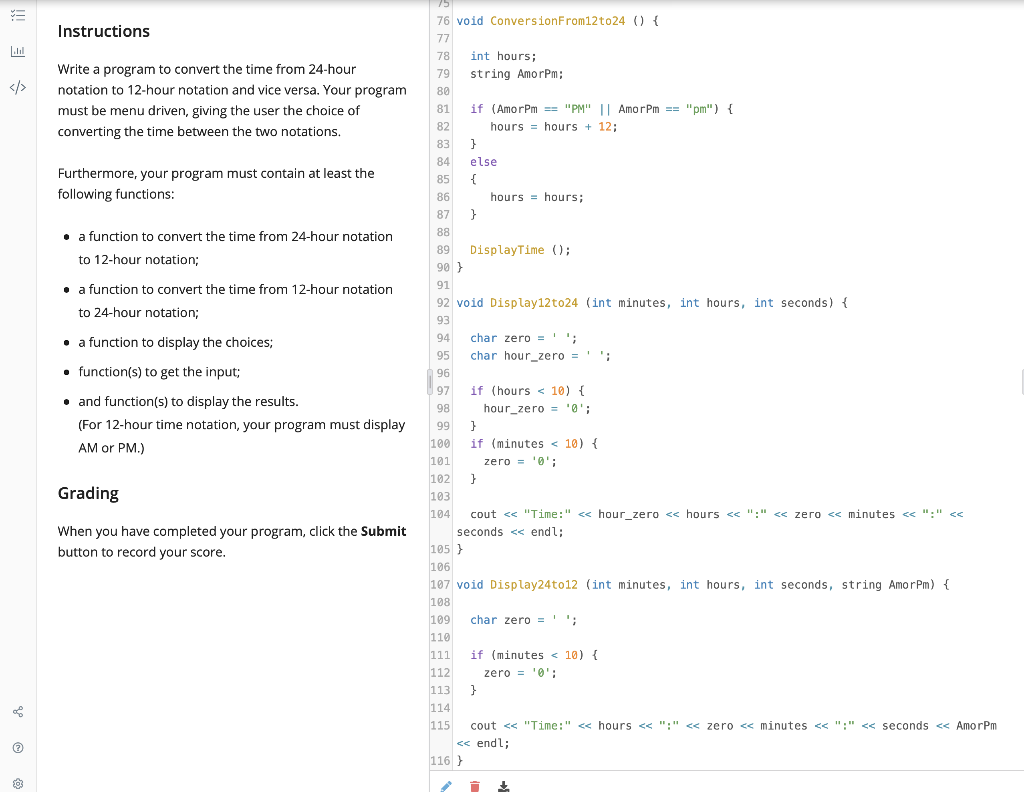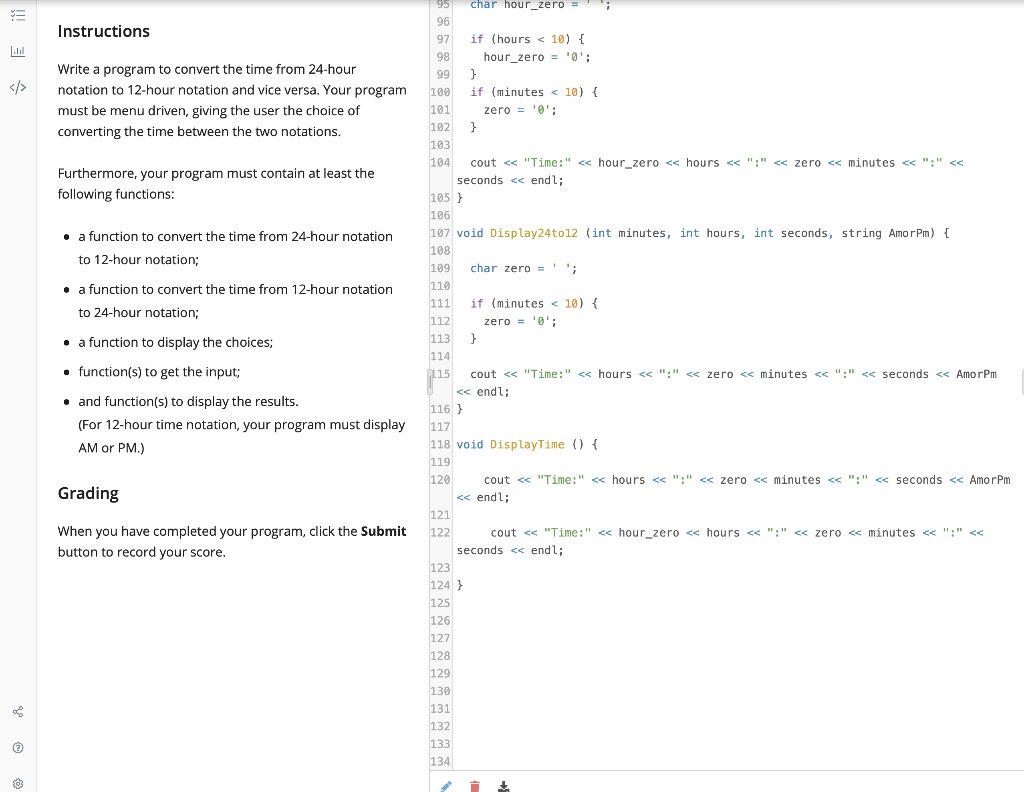Need some assistance of reorganizing this whole program. I have the right code for everything I just need help on putting all the codes in the right spot so it can come out to the correct output.

output is supposed to look like this:

1 \\ user inputs choice to convert 12 to 24

8 \\ user inputs 8 for hours

30 \\ user inputs 30 for minutes

20 \\ user inputs 20 for seconds

AM \\ user inputs AM for morning time

8:30:20 \\ then program displays the time converted

99 \\ user inputs 99 to exit the program

v +include <iostreams 2 #include <string> Instructions 3 Write a program to convert the time from 24-hour notation to 12-hour notation and vice versa. Your program must be menu driven, giving the user the choice of converting the time between the two notations. Furthermore, your program must contain at least the following functions: . a function to convert the time from 24-hour notation to 12-hour notation; • a function to convert the time from 12-hour notation to 24-hour notation; • a function to display the choices; • function(s) to get the input; • and function(s) to display the results. (For 12-hour time notation, your program must display AM or PM.) 4 using namespace std; 5 6 void DisplayChoices (); 7 void getTime (int minutes, int hours, int seconds); 8 void ConversionFrom12to24 (; 9 void ConversionFrom24t012 (); 10 void DisplayTime (); 11 12 int main() { 13 14 int input = ''; 15 DisplayChoices (); 16 cin >> input; 17 18 while (input ) { 19 20 switch (input) { 21 22 case 'l': ConversionFrom12to24 (); 23 break; 24 25 case '2': ConversionFrom24t012 (); 26 break; 27 28 case '99': 29 30 31 32 33 return; 34 35} 36 37 void DisplayChoices () { 38 39 cout << "press 1 to convert from 12-hour to 24-hour notation or << endl; 40 cout << "press 2 to convert form 24-hour to 12-hour notation" << endl; 41 cout << "Enter 99 to exit the program" << endl; 42 43} 44 void getTime (int minutes, int hours, int seconds, string AmorPm) { Grading } } When you have completed your program, click the Submit button to record your score.
v Instructions Write a program to convert the time from 24-hour notation to 12-hour notation and vice versa. Your program must be menu driven, giving the user the choice of converting the time between the two notations. Furthermore, your program must contain at least the following functions: . a function to convert the time from 24-hour notation to 12-hour notation; • a function to convert the time from 12-hour notation to 24-hour notation; • a function to display the choices; • function(s) to get the input; 37 void DisplayChoices (){ 38 39 cout << "press 1 to convert from 12-hour to 24-hour notation or << endl; 40 cout << "press 2 to convert form 24-hour to 12-hour notation" << endl; 41 cout << "Enter 99 to exit the program" << endl; 42 43] 44 void getTime (int minutes, int hours, int seconds, string AmorPm) { 45 46 cout << "Time in hours: "; 47 cin >> hours: 48 49 cout << "Time in minutes: "; 50 cin >> minutes; 51 52 cout << "Time in seconds: "; 53 cin >> seconds; 54 55 cout << "AM or PM?"; 56 cin >> Amor Pm; 57 } 58 59 void ConversionFrom24t012 () { 60 61 int hours; 62 string Amor Pm = "; 63 64 if (hours <= 12) { 65 AmorPm = "AM"; 66 } 67 else if (hours > 12) { 68 Amor Pm = "PM"; 69 hours = hours % 12; 70 } 71 72 DisplayTime (); 73 74} 75 76 void ConversionFrom12to24 () { 77 78 int hours; 79 string AmorPm; • and function(s) to display the results. (For 12-hour time notation, your program must display AM or PM.) Grading When you have completed your program, click the Submit button to record your score.
v Instructions Write a program to convert the time from 24-hour notation to 12-hour notation and vice versa. Your program must be menu driven, giving the user the choice of converting the time between the two notations. } Furthermore, your program must contain at least the following functions: { } . a function to convert the time from 24-hour notation to 12-hour notation; • a function to convert the time from 12-hour notation to 24-hour notation; • a function to display the choices; • function(s) to get the input; 75 76 void ConversionFrom12to24 () { 77 78 int hours; 79 string Amor Pm; 80 81 if (Amor Pm == "PM" || Amor Pm == "m") { 82 hours = hours + 12; 83 84 else 85 86 hours = hours; 87 88 89 DisplayTime(); 90 } 91 92 void Display12to24 (int minutes, int hours, int seconds) { 93 94 char zero = ''; 95 char hour_zero = ''; 96 97 if (hours < 10) { 98 hour_zero = '0'; 99 } 100 if (minutes < 10) { 101 zero = '0'; 102 103 104 cout << "Time:" << hour_zero << hours << ":" << zero << minutes « "." << seconds << endl; 105} 106 107 void Display24to12 (int minutes, int hours, int seconds, string Amor Pm) { 108 109 char zero = ''; 110 111 if (minutes < 10) { 112 zero = ''; 113 } 114 115 cout << "Time:" << hours << ":" << zero < minutes <<":" << seconds << Amorpm << endl; 116 } • and function(s) to display the results. (For 12-hour time notation, your program must display AM or PM.) } Grading When you have completed your program, click the Submit button to record your score.
v Instructions } Write a program to convert the time from 24-hour notation to 12-hour notation and vice versa. Your program must be menu driven, giving the user the choice of converting the time between the two notations. Furthermore, your program must contain at least the following functions: . a function to convert the time from 24-hour notation to 12-hour notation; • a function to convert the time from 12-hour notation to 24-hour notation; • a function to display the choices; 113 • function(s) to get the input; 95 char hour_zero- 96 97 if (hours < 10) { 98 hour_zero = '0'; 99 100 if (minutes < 10) { 101 zero = '0'; 102 } 103 104 cout << "Time:" << hour_zero << hours << ":" << zero << minutes << ":" << seconds << endl; 105) 106 107 void Display24to12 (int minutes, int hours, int seconds, string Amor Pm) { 108 109 char zero = ''; 119 111 if (minutes < 10) { 112 zero = ''; } 114 L15 cout << "Time:" << hours <<":"« zero << minutes << ":" << seconds << Amor Pm << endl; 116 } 117 118 void DisplayTime () { 119 120 cout << "Time:" << hours << ":" << zero << minutes << ":" << seconds << Amor Pm << endl; 121 122 cout << "Time:" << hour_zero << hours << ":" « zero « minutes << ":" « seconds << endl; 123 124 } 125 126 127 128 129 130 131 132 133 134 • and function(s) to display the results. (For 12-hour time notation, your program must display AM or PM.) Grading When you have completed your program, click the Submit button to record your score.

#### Homework Answers

Answer #1

Note: For any issues or doubts, just put a comment before giving a thumbs down. Thanks.

COde( There were many faults in the logic so I fixed them ):

#include <iostream>
#include <string>

using namespace std;

void DisplayChoices();
void getTime12Format();
void getTime24Format();
void ConversionFrom12to24(int minutes, int hours, int seconds, string AMorPM);
void ConversionFrom24to12(int minutes, int hours, int seconds);
void Display12to24(int minutes, int hours, int seconds);
void Display24to12(int minutes, int hours, int seconds, string AMorPM);

int main(){

int input;
DisplayChoices();
cin>> input;

switch (input)
{
case 1:
getTime12Format();
break;

case 2:
getTime24Format();
break;

case 99:
exit(0);

default:
cout<<"Invalid Option!!";
break;
}

return 0;
}

void DisplayChoices(){
cout<< "press 1 to convert from 12-hour to 24-hour notation or"<<endl;
cout<< "press 2 to convert from 24-hour to 12-hour notation"<<endl;
cout<< "Enter 99 to exit the program"<<endl;
}

void getTime12Format(){
int hours;
cout<< "Time in hours: ";
cin>> hours;

int minutes;
cout<< "Time in minutes: ";
cin>> minutes;

int seconds;
cout<< "Time in seconds: ";
cin>> seconds;

string AMorPM;
cout<< "AM or PM: ";
cin>>AMorPM;
ConversionFrom12to24(minutes, hours, seconds, AMorPM);
}

void getTime24Format(){
int hours;
cout<< "Time in hours: ";
cin>> hours;

int minutes;
cout<< "Time in minutes: ";
cin>> minutes;

int seconds;
cout<< "Time in seconds: ";
cin>> seconds;
ConversionFrom24to12(minutes, hours, seconds);
}

void ConversionFrom24to12(int minutes, int hours, int seconds){
string AMorPM = "";

if(hours>12){
AMorPM = "PM";
hours = hours%12;
}
else
{
AMorPM = "AM";
hours = hours;
}

Display24to12(minutes, hours, seconds, AMorPM);
}

void ConversionFrom12to24(int minutes, int hours, int seconds, string AmorPm){

if(AmorPm == "PM"){
hours = hours + 12;
}
else
{
hours = hours;
}

Display12to24(minutes, hours, seconds);
}

void Display12to24(int minutes, int hours, int seconds){
char zero = ' ';
char hour_zero = ' ';

if(hours < 10){
hour_zero = '0';
}
if(minutes < 10)
{
zero = '0';
}

cout<<"Time: "<<hour_zero<<hours<<":"<<zero<<minutes<<":"<<seconds<<endl;

}

void Display24to12(int minutes, int hours, int seconds, string AMorPM){
char zero = ' ';

if(minutes<10){
zero = '0';
}

cout<<"Time: "<<hours<<":"<<zero<<minutes<<":"<<seconds<<AMorPM<<endl;
}

Know the answer?
Your Answer:

#### Post as a guest

Your Name:

What's your source?

#### Earn Coin

Coins can be redeemed for fabulous gifts.

Not the answer you're looking for? Ask your own homework help question. Our experts will answer your question WITHIN MINUTES for Free.
Similar Homework Help Questions
• ### 1 Write a program to convert the time from 24-hour notation to 12-hour notation and vice...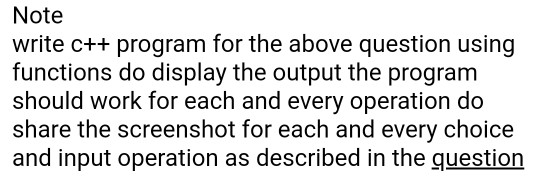1 Write a program to convert the time from 24-hour notation to 12-hour notation and vice versa. Your program must be menu driven, giving the user the choice of converting the time between the two notations. Furthermore, your program must contain at least the following functions: a function to convert the time from 24-hour notation to 12-hour notation, a function to convert the time from 12-hour notation to 24-hour notation, a function to display the choices, function(s) to get the...

• ### Write in C++ please In Chapter 10, the class clockType was designed to implement the time...

Write in C++ please In Chapter 10, the class clockType was designed to implement the time of day in a program. Certain applications, in addition to hours, minutes, and seconds, might require you to store the time zone. Derive the class extClockType from the class clockTypeby adding a member variable to store the time zone. Add the necessary member functions and constructors to make the class functional. Also, write the definitions of the member functions and the constructors. Finally, write...

• ### This is a c++ program. Use the description from Parking Ticket Simulator (listed below) as a basis, where you need to...

This is a c++ program. Use the description from Parking Ticket Simulator (listed below) as a basis, where you need to create a Car class and Police Officer class, to create a new simulation. Write a simulation program (refer to the Bank Teller example listed below) that simulates cars entering a parking lot, paying for parking, and leaving the parking lot. The officer will randomly appear to survey the cars in the lot to ensure that no cars are parked...

• ### I have a program that needs comments added to it: #include <iostream> #include <stdio.h> #include <conio.h>...

I have a program that needs comments added to it: #include <iostream> #include <stdio.h> #include <conio.h> #include <windows.h> using namespace std; int main() { int m, s,h; cout << "A COUNTDOWN TIMER " << endl; cout << "enter time in hours here" << endl; cin >> h; cout << "enter time in minutes here " << endl; cin >> m; cout << "enter time in seconds here" << endl; cin >> s; cout << "Press any key to start" <<...

• ### I need help modifying this code please ASAP using C++ Here is what I missed on this code below Here are the instruction...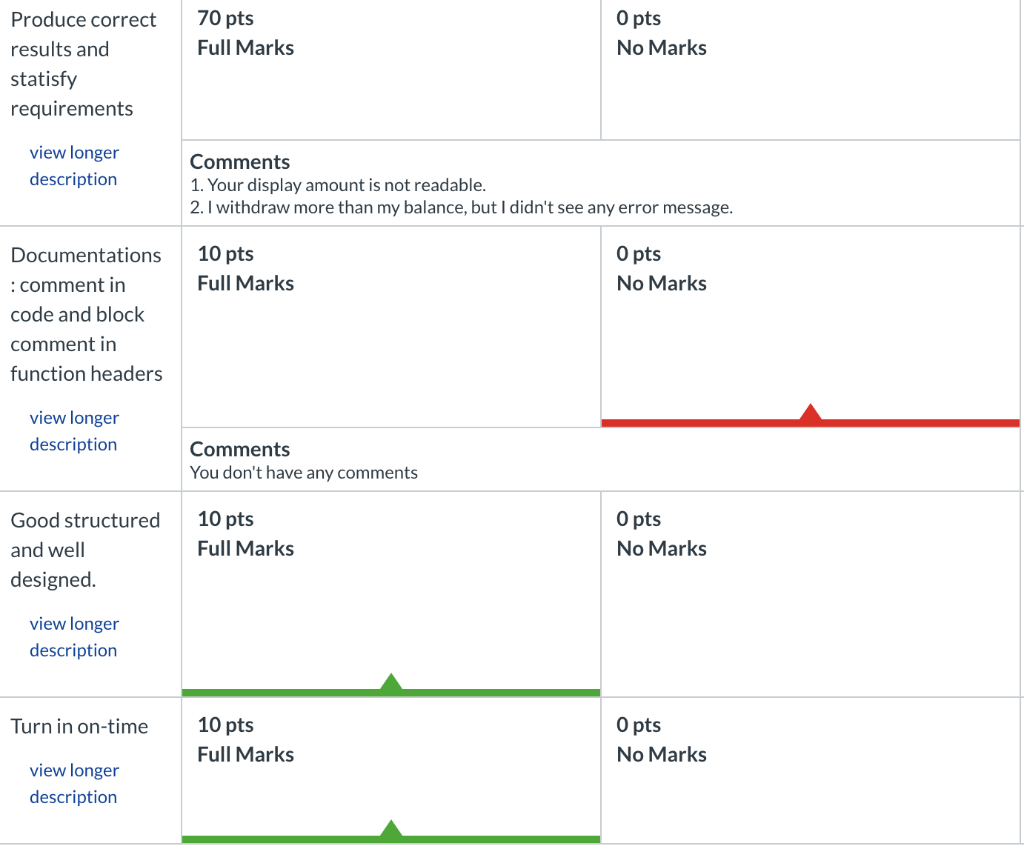I need help modifying this code please ASAP using C++ Here is what I missed on this code below Here are the instructions Here is the code Produce correct70 pts O pts Full Marks No Marks results and statisfy requirements view longer Comments 1. Your display amount is not readable 2. I withdraw more than my balance, but I didn't see any error message description Documentations10 pts 0 pts Full Marks No Marks : comment i code and block comment...

• ### so i have my c++ code and ive been working on this for hours but i...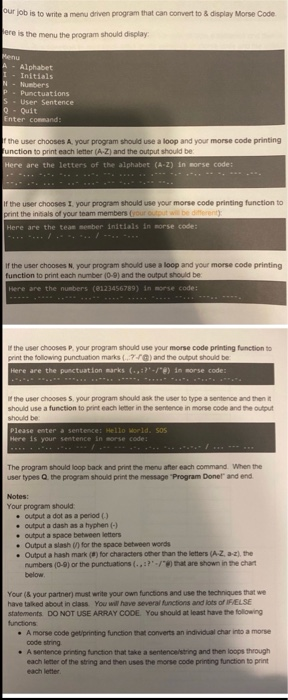so i have my c++ code and ive been working on this for hours but i cant get it to run im not allowed to use arrays. im not sure how to fix it thank you for the help our job is to write a menu driven program that can convert to display Morse Code ere is the menu the program should display Menu Alphabet Initials N-Numbers - Punctuations S = User Sentence Q- Quit Enter command the user chooses...

• ### I need to make a few changes to this C++ program,first of all it should read...

I need to make a few changes to this C++ program,first of all it should read the file from the computer without asking the user for the name of it.The name of the file is MichaelJordan.dat, second of all it should print ,3 highest frequencies are: 3 words that occure the most.everything else is good. #include <iostream> #include <map> #include <string> #include <cctype> #include <fstream> #include <iomanip> using namespace std; void addWord(map<std::string,int> &words,string s); void readFile(string infile,map<std::string,int> &words); void display(map<std::string,int>...

• ### I need help with a program. My program wont run. I cant fix the errors. Its...

I need help with a program. My program wont run. I cant fix the errors. Its mainly c++. I am trying to run it in dev c++. if anyone can fix this program i will be glad. #include <iostream> #include <fstream> #include <string> #include <vector> #include <map> #include <iomanip> #include <algorithm> #include <stdlib.h> #include <time.h> using namespace std; bool buildMap(); void writeIndex(); void AddWordtoMap(string); void writeSorted(); void Filter(); map <string, int> jobMap; map <string, int> filterMap; typedef pair<string, int> myPair;...

• ### C++ Program Beginner Program with Struct

Hello there, I am trying to write a program that prompts the user for the hour, minutes and seconds for a 24-hour time(military time). If the time is valid then onesecond is added to it, and new time is output to the screen.Here are some of my class notes...I should be including.struct TimeDeclare a struct named: TimeThe struct has 3 data members: hours, minutes and seconds. (all integers)In main(), declare and object of Time and name it: timeFour functions in...

• ### C++ Program Beginner Program with Struct

Hello there, I am trying to write a program that prompts the user for the hour, minutes and seconds for a 24-hour time(military time). If the time is valid then onesecond is added to it, and new time is output to the screen.Here are some of my class notes.I should be including.struct TimeDeclare a struct named: TimeThe struct has 3 data members: hours, minutes and seconds. (all integers)In main(), declare and object of Time and name it: timeFour functions in...